## The role of nuclear fission in the nucleosynthesis of the rapid neutron capture processLA-UR-19-22694

### Matthew Mumpower

LBNL Theory Seminar

Wednesday April 3$^{rd}$ 2019FIRE Collaboration
Fission In R-process Elements

## The answer to this question requiresKnowledge of astrophysical conditions (variations in current simulations)

Knowledge of nuclear physics inputs (1000's of unknown species / properties)

(Both are needed to model the nucleosynthesis)

And precise observations!

In other words, the solution is quite difficult...

Beers & Christlieb ARAA (2005) • Metzger et al. MNRAS (2010) • Barnes et al. ApJ (2016) • Côté et al. ApJ (2018)

## Schematic of a kilonova

Two component model: wind & dynamical ejectRed emission: late (weeks); lanthanide dominated

blue emission: early (days); UV dominated

Metzger et al. MNRAS 406 4 (2010) • Kasen et al. Nature 551 80 (2017) • Figure from Korobkin et al. (2019)

## Nuclear physics as the language of the $r$-process

1st order: masses, $\beta$-decay rates, capture rates & fissionDillmann et al. EPJA (2002) • Aprahamian et al. (2018) • See review paper: Mumpower et al. PPNP 86 (2016)

## What do we know?

The chart of nuclides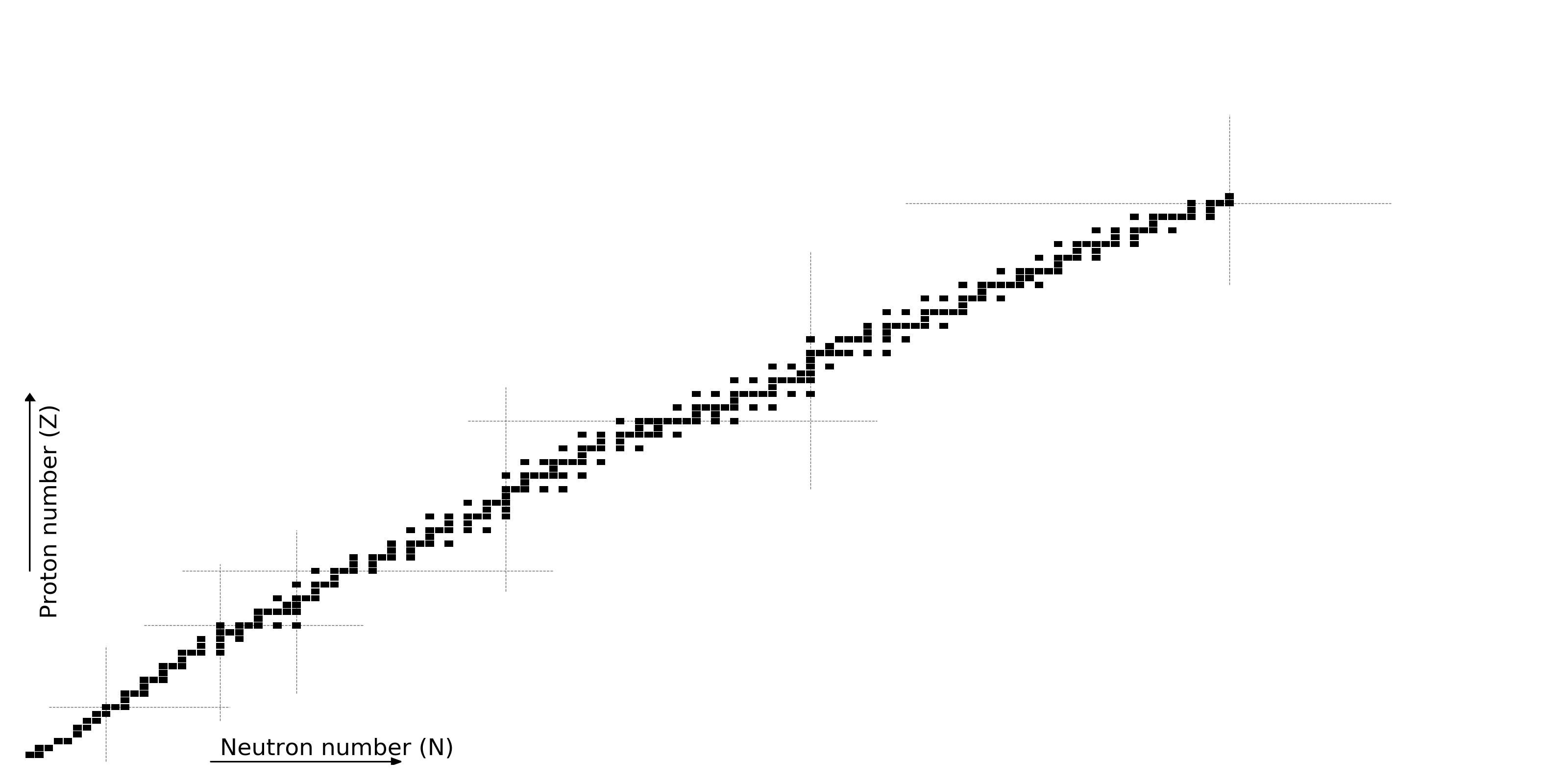Figure by M. Mumpower

## What do we know?

All half-lives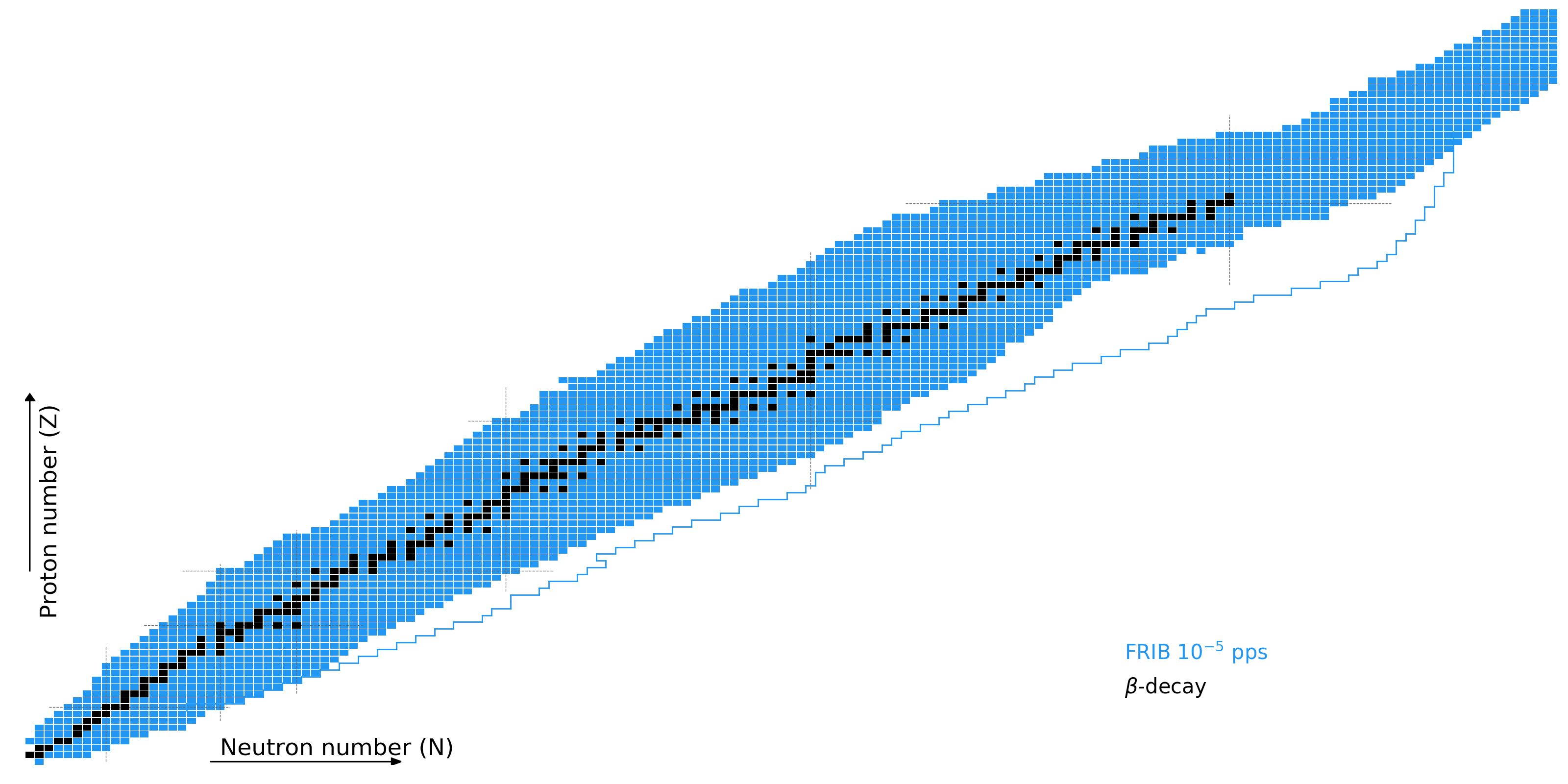Figure by M. Mumpower

## What do we know?

Recently measured beta-decay half-lives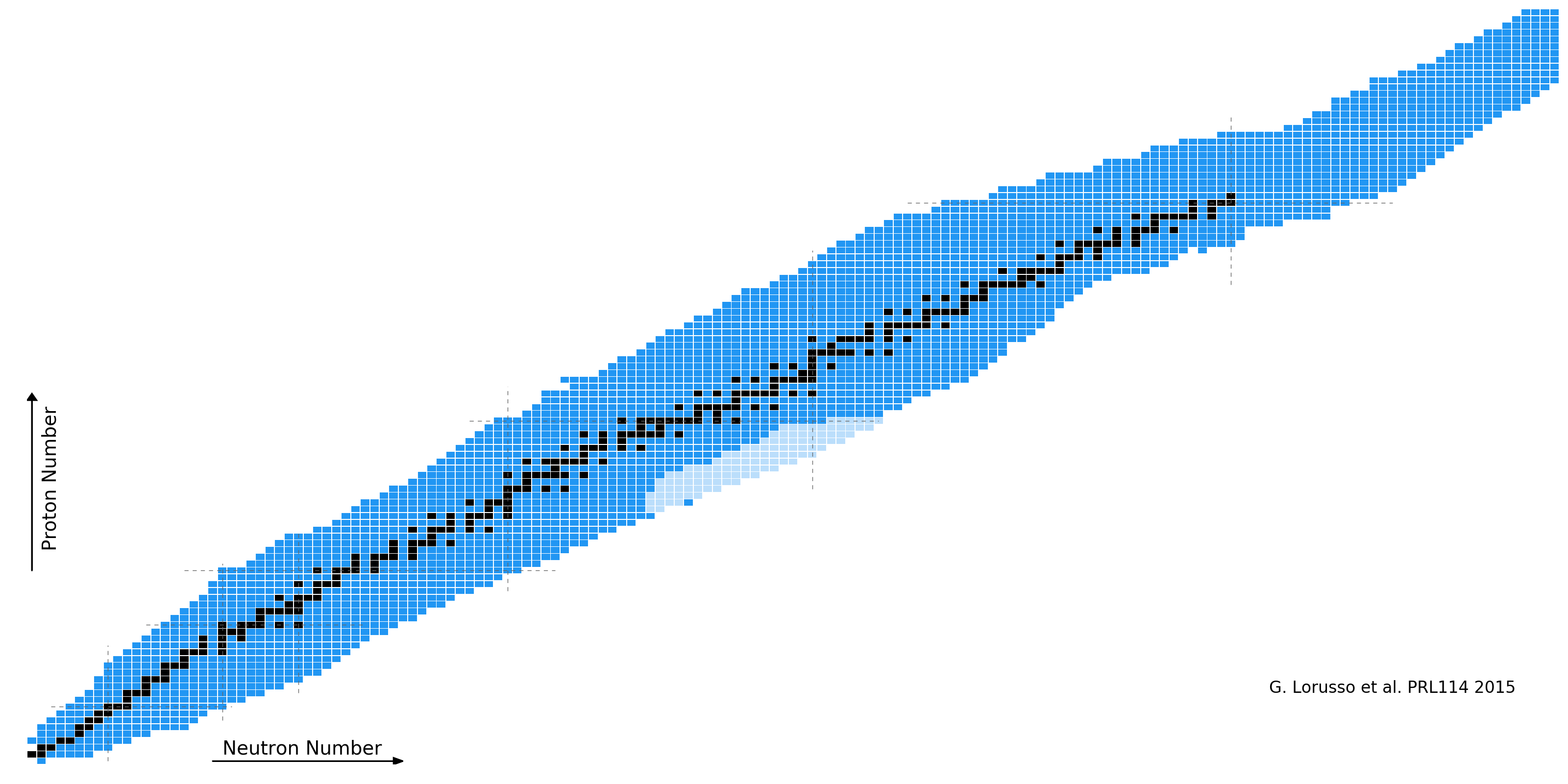Figure by M. Mumpower

## What do we know?

Recently measured beta-decay half-lives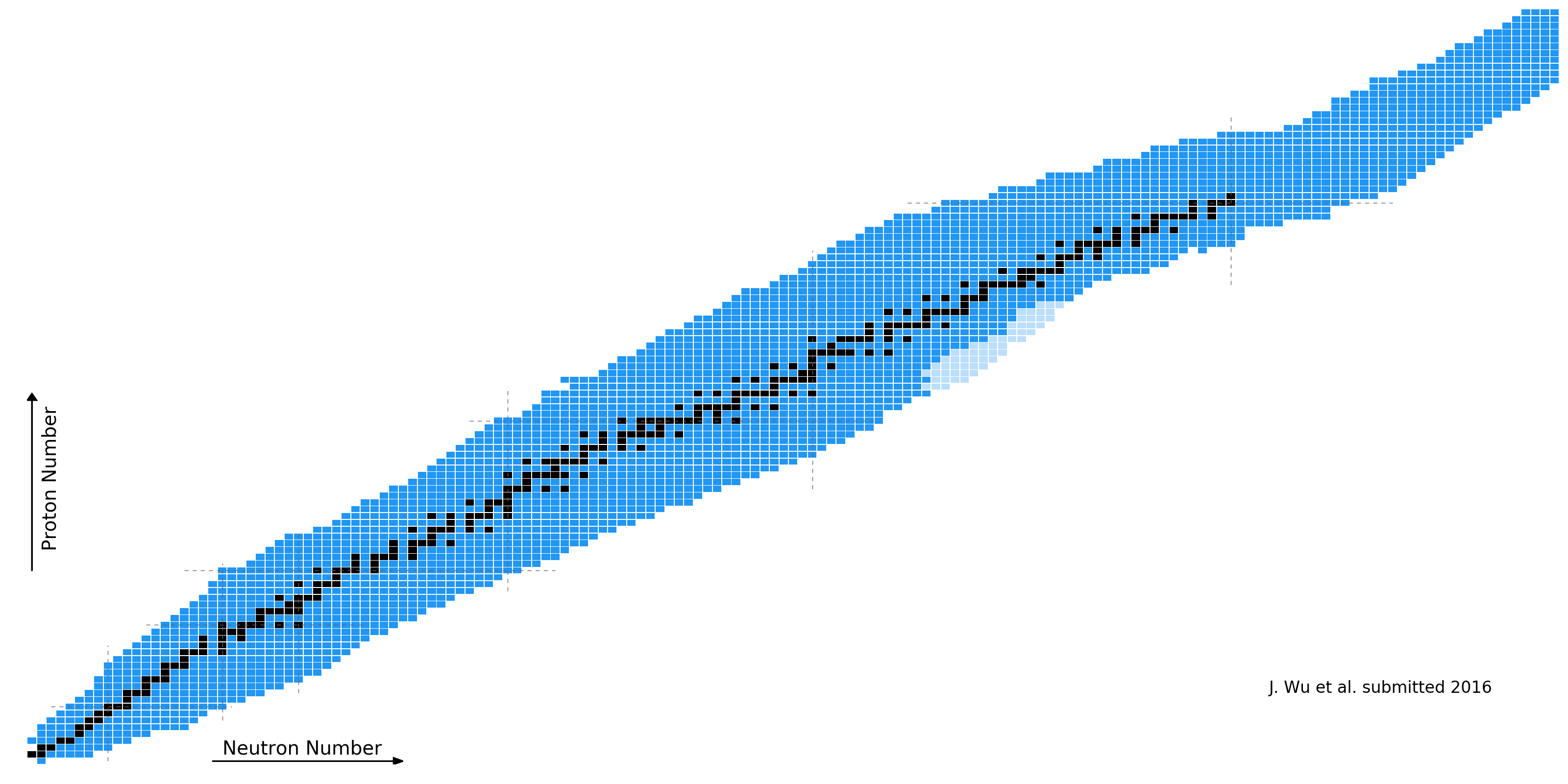Figure by M. Mumpower

## What do we know?

Nuclear masses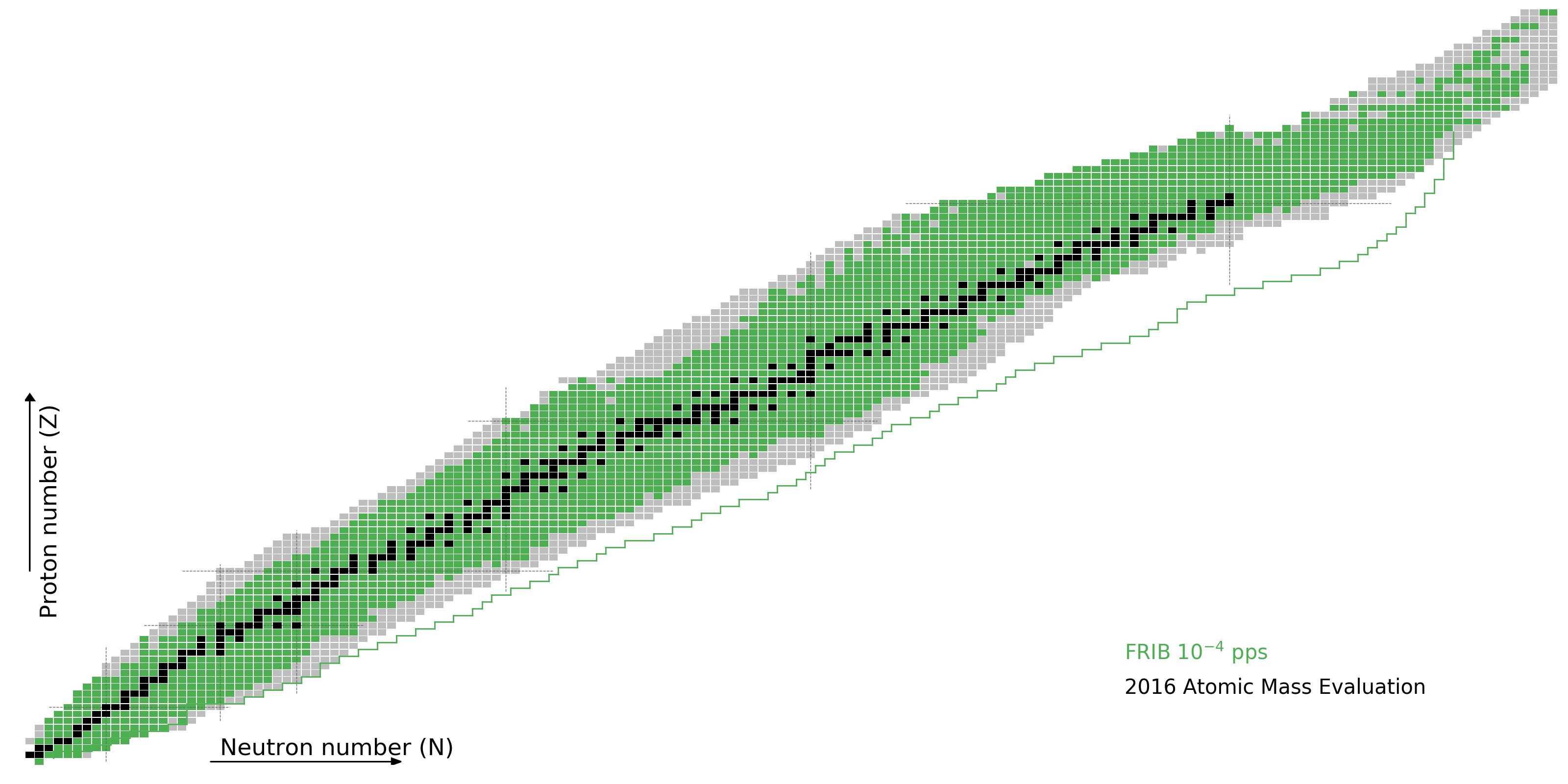Figure by M. Mumpower

## What do we know?

Neutron capture rates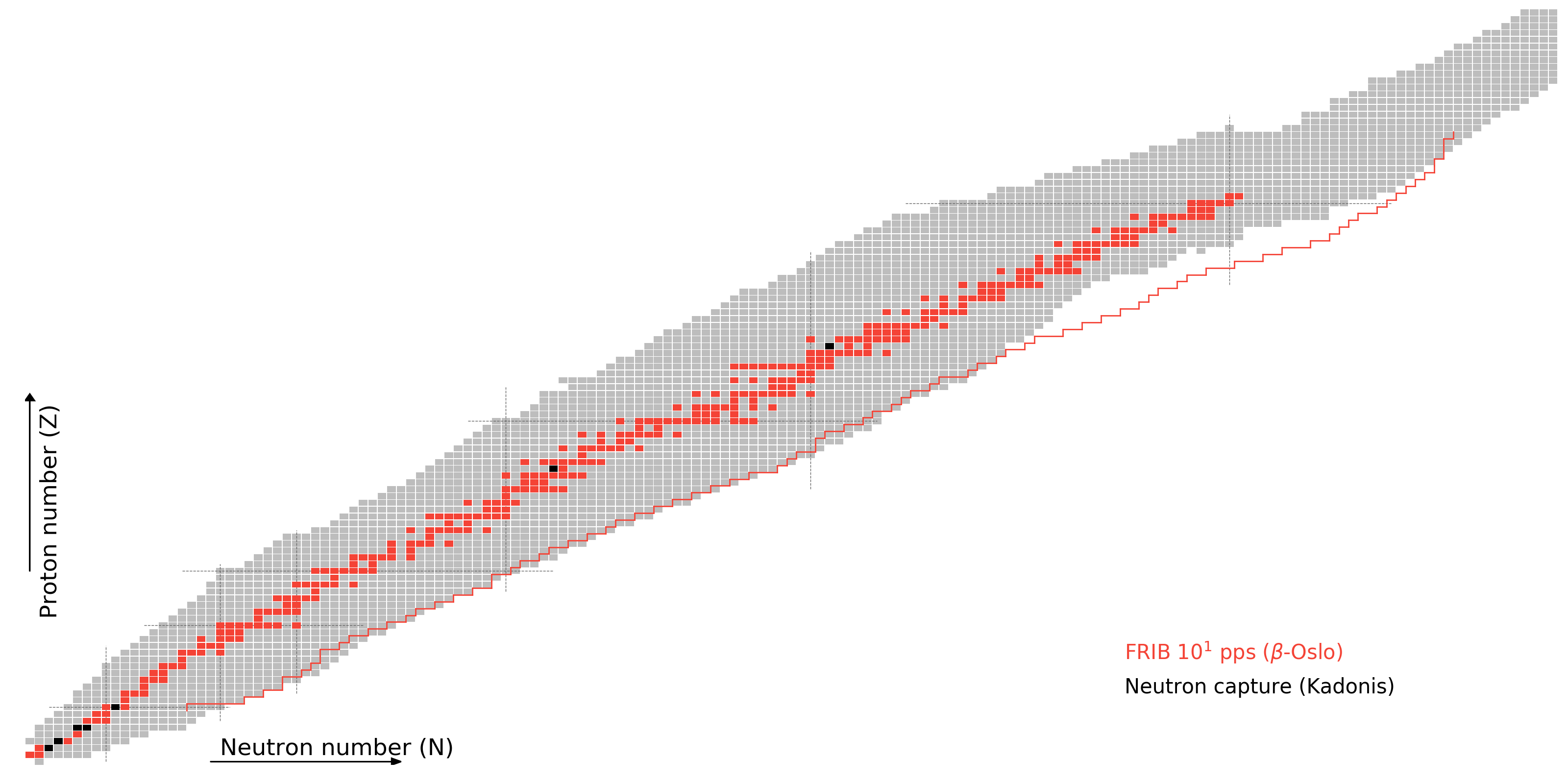Figure by M. Mumpower

## What do we know?

As of today, to varying degrees of accuracyFigure by M. Mumpower

## Much will be measured at FRIB

But fission studies will remain relatively inaccessible∴ Fission theory is critical find any sort of "smoking gun" of heavy element production

Spyrou et al. PRL (2016) • Vilen et al. • PRL (2018) Orford et al. PRL (2018) • Sprouse et al. (2019) • Figure by Mumpower

## Nuclear fission in a nutshell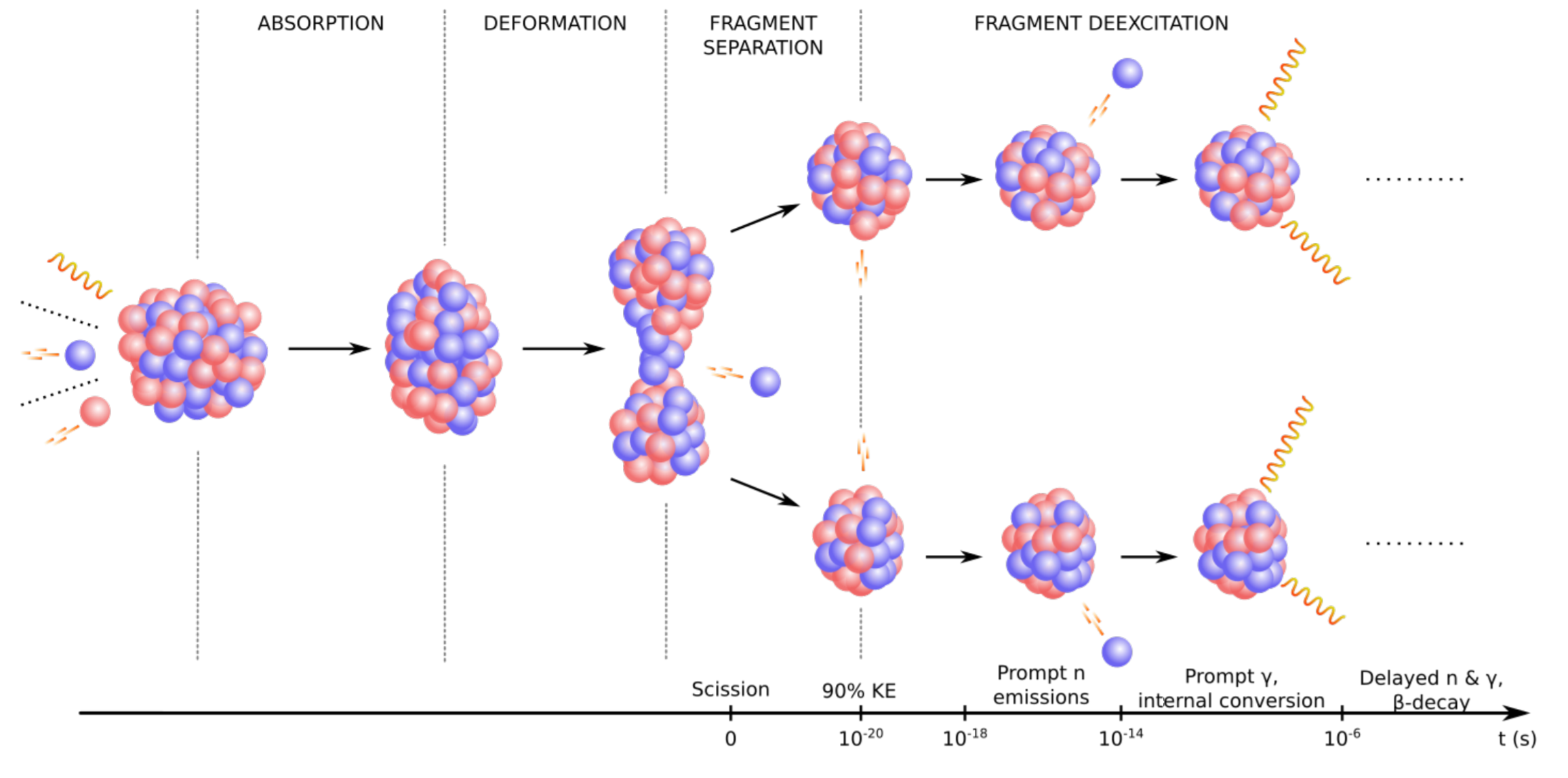Influence on the $r$-process:

Fission rates and branching determine re-cycling (robustness)

Fragment yields place material at lower mass number; barriers determine hot spots

Large Q-value ⇒ impacts thermalization and therefore possibly observations

Responsible for what is left in the heavy mass region when nucleosynthesis is complete ⇒ "smoking gun"

Meitner & Frisch (1938) • Bohr & Wheeler (1939) • Figure from Verriere & Mumpower in prep. (2019)

## Estimated Barrier Heights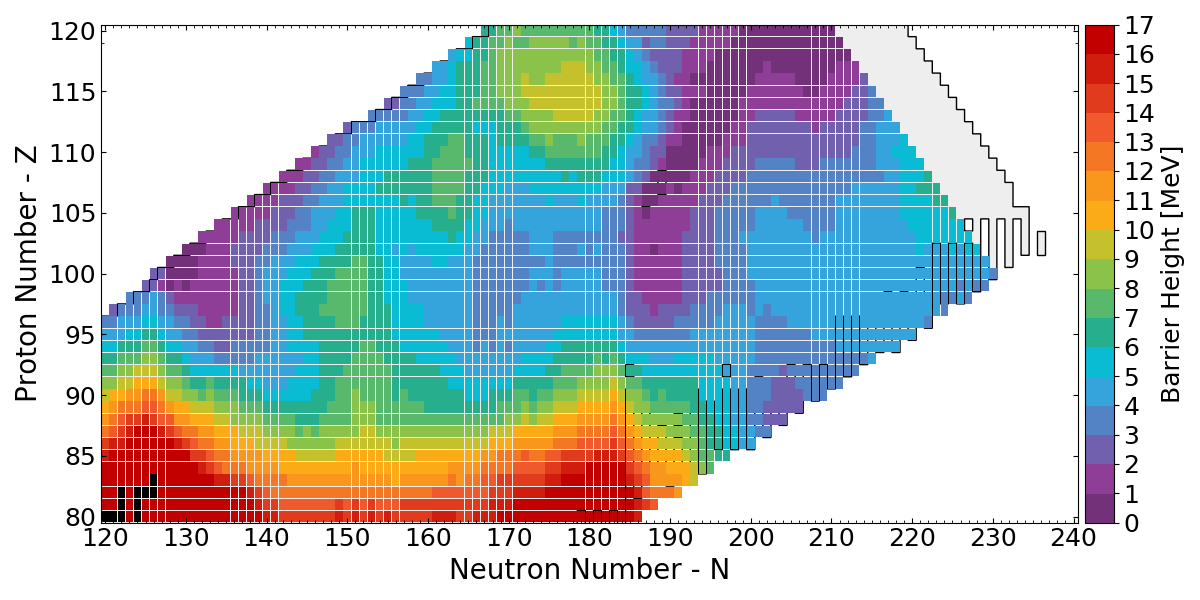FRLDM Barrier heights

Low barrier ↦ fission ⇑

$r$-process hot spots follow low barriers

Möller et al. PRC 91 024310 (2015) • Vassh et al. J. Phys. G (2019)

## Neutron-induced fission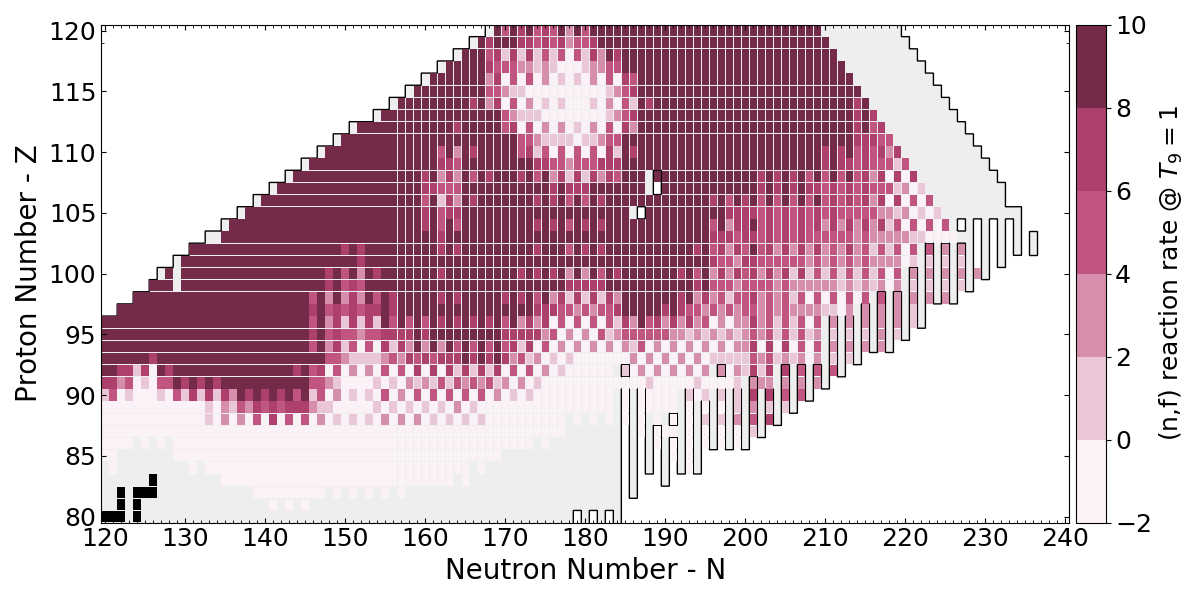Toshihiko's CoH statistical Hauser-Feshbach

Barrier heights Möller (2015) / FRDM2012 masses

Large region that will fission cycle $r$-process

J. Verbeke, J. Randrup, R. Vogt Comp. Phys. Comm. 191 178 (2015) • Mumpower et al. ApJ 869 1 (2018) • Vassh et al. J. Phys. G (2019)

## Modeling $\beta$ -delayed fission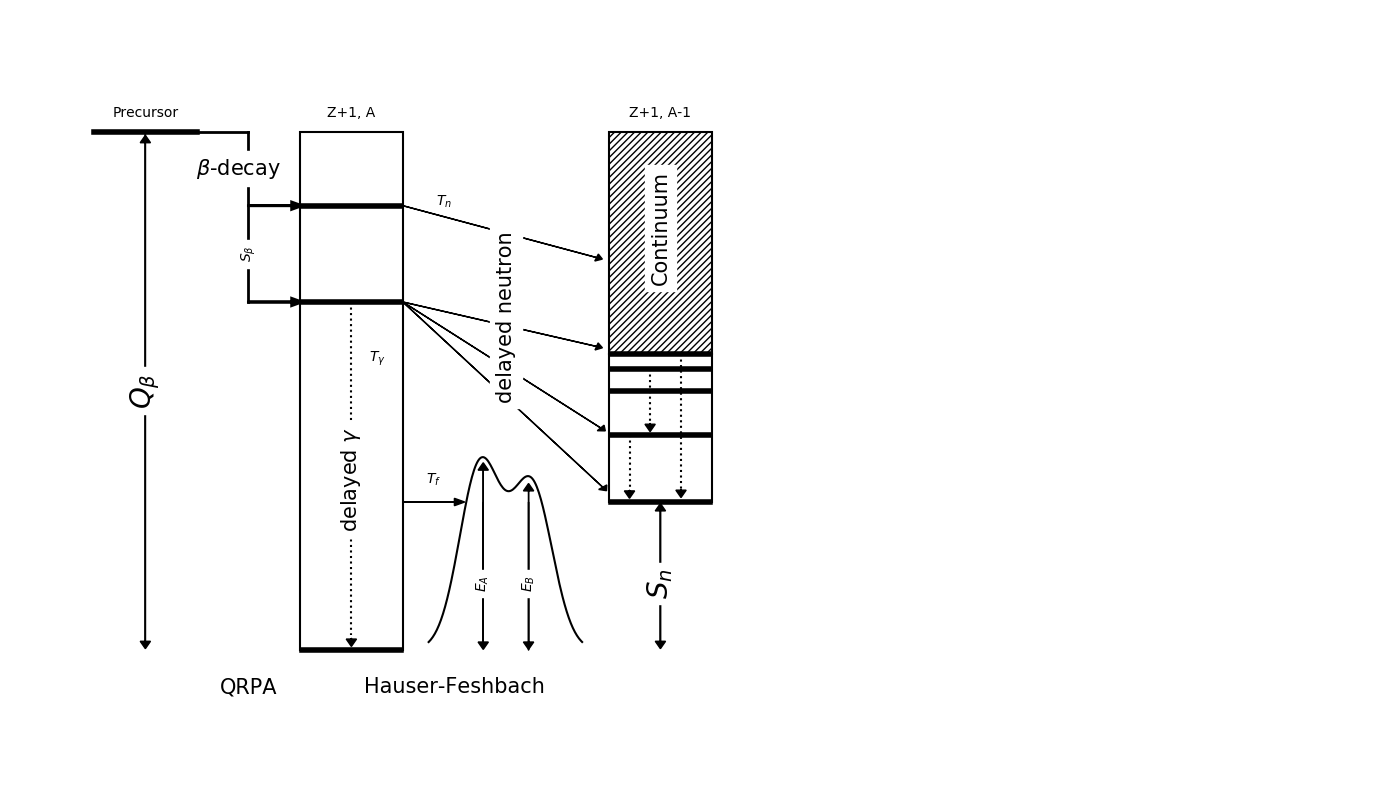We have a model to describe nuclear de-excitation called QRPA+HF

We have recently extended the our QRPA+HF model to describe $\beta$-delayed fission ($\beta$df)

Barrier heights from Möller et al. PRC 91 024310 (2015)

Kodama & Takahashi (1975) • Thielemann et al. (1983) • Shibagaki et al. ApJ 816 2 (2016) • Mumpower et al. ApJ 869 1 (2018)

## Multi-chance $\beta$ dfNear the dripline $Q_{beta}$ ⇡ $S_{n}$ ⇣

Multi-chance $\beta$df: each daughter may fission

New fission channel to consider for $r$-process calculations

The yields in this decay mode are a convolution of many fission yields!

Mumpower et al. ApJ 869 1 (2018) • Mumpower et al. in prep (2019)

## ($n$,$\gamma$,$f$) competition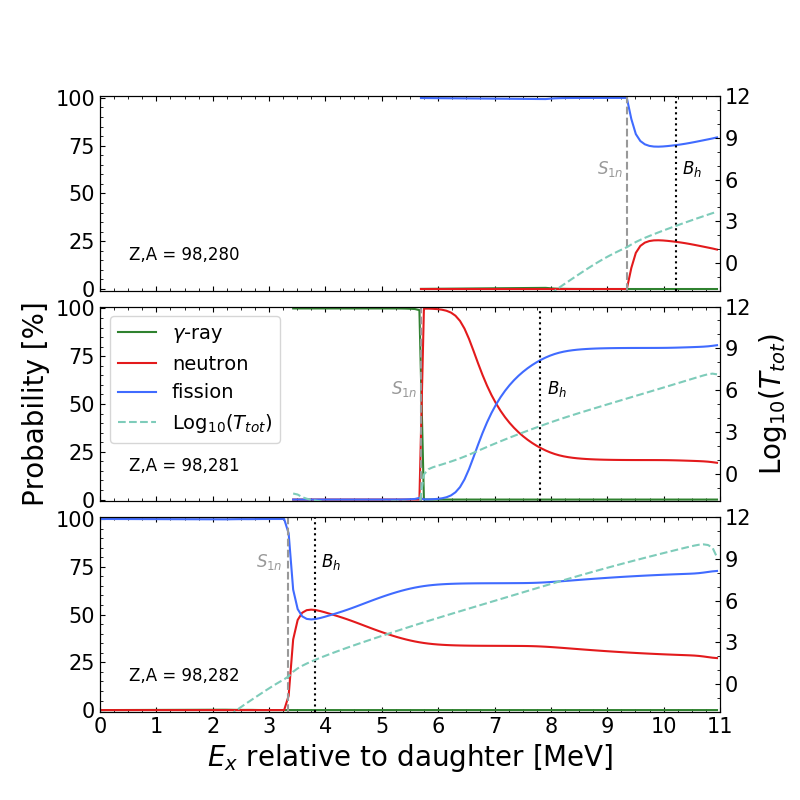Fission can successfully compete with $\gamma$-rays and neutrons

Particle spectra also produced which are of interest for observations

Mumpower et al. PRC (2016) • Mumpower et al. ApJ 869 1 (2018) • Möller et al. ADNDT 125 (2019) • Mumpower et al. in prep (2019)

## Cumulative $\beta$ df probability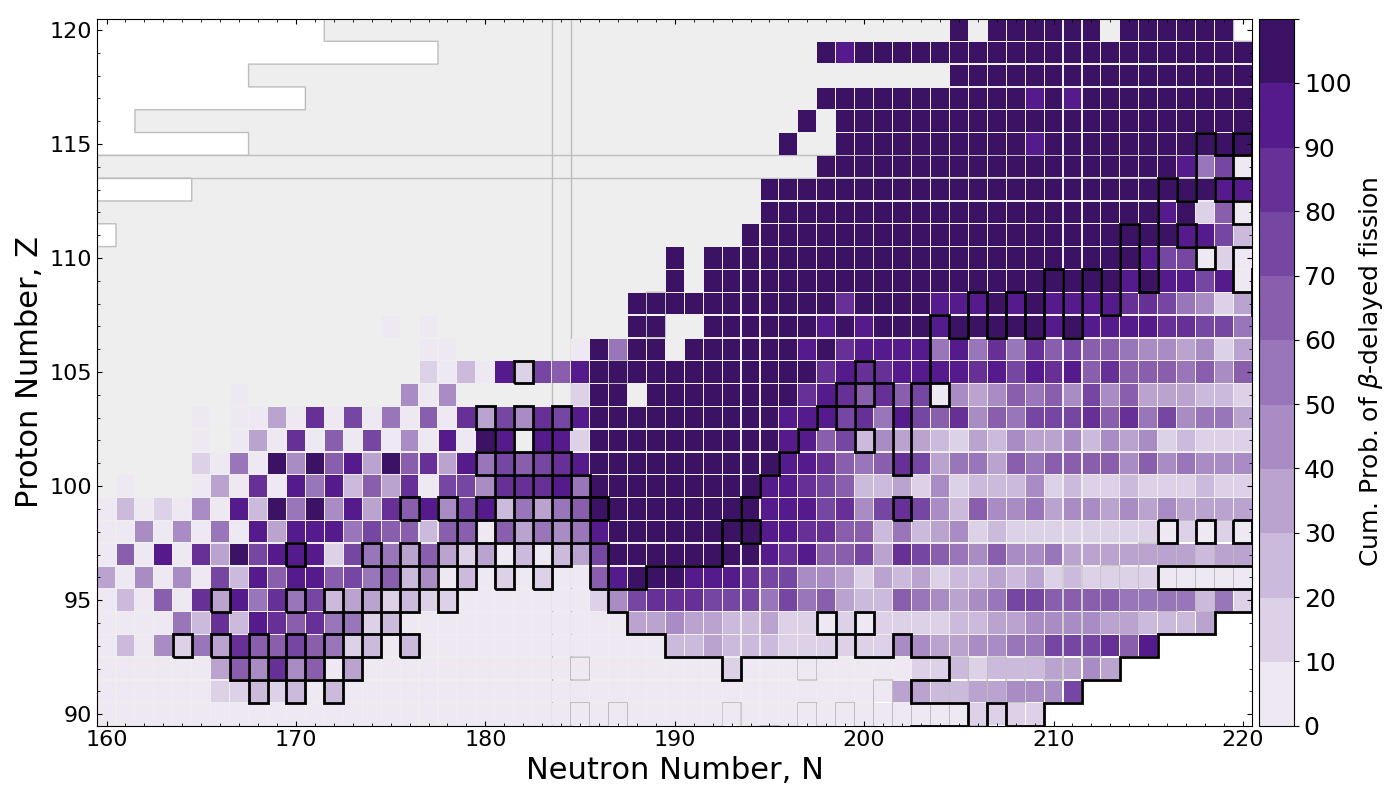$\beta$df occupies a large amount of real estate in the NZ-plane

Multi-chance $\beta$df outlined in black

Mumpower et al. ApJ 869 1 (2018) • Mumpower et al. in prep (2019)

## Impact on final abundances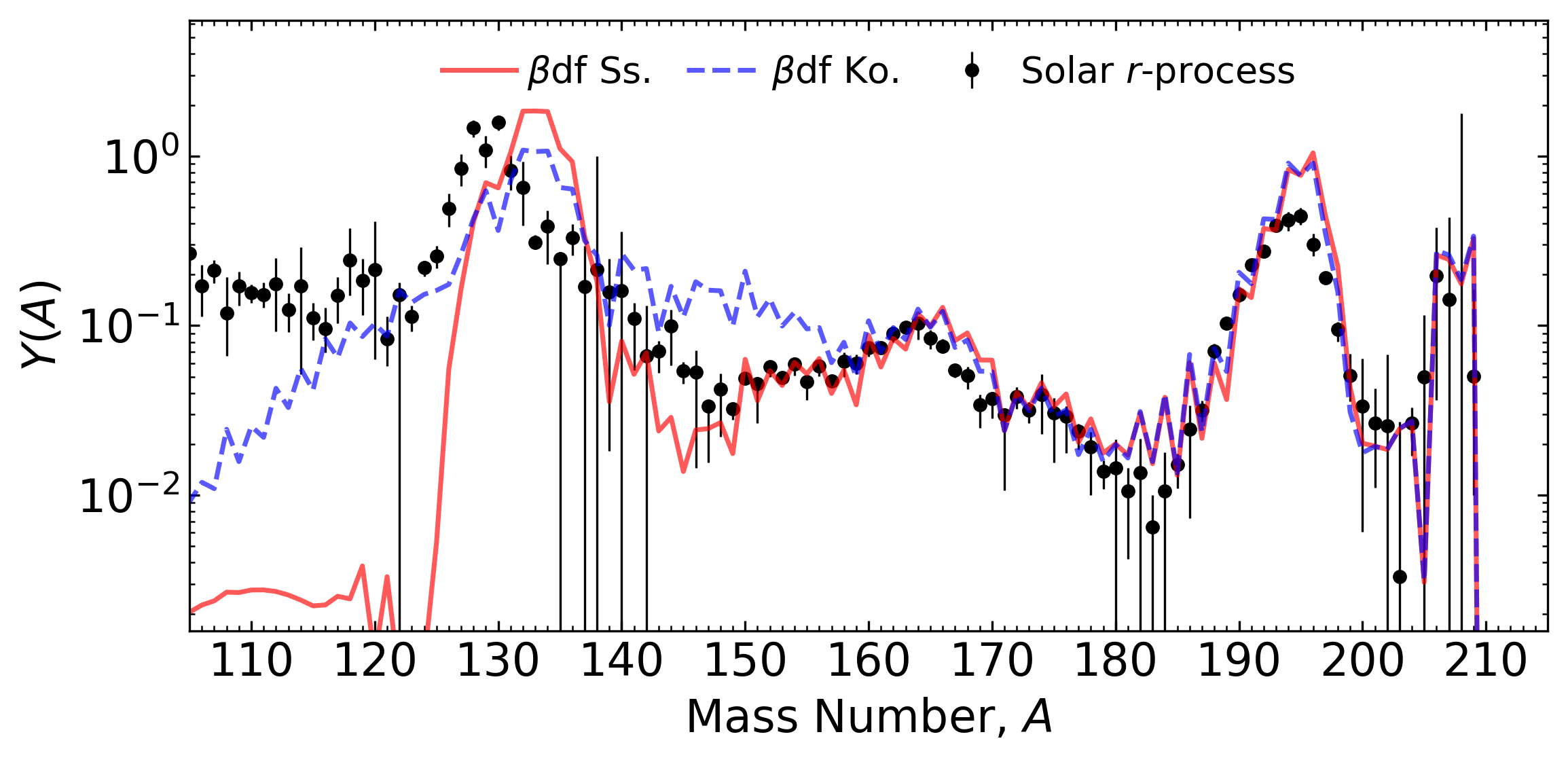Network calculation of tidal ejecta from a neutron star merger (FRDM2012)

$\beta$df can shape the final pattern near the $A=130$ peak

This is because of a relatively long fission timescale

Conclusion ⇒ we need a good description of fission yields to understand abundances near $A\sim130$.

Mumpower et al. ApJ 869 1 (2018) • Möller, Mumpower et al. ADNDT (2019) • Mumpower et al. in prep (2019)

## A second consequence of $\beta$df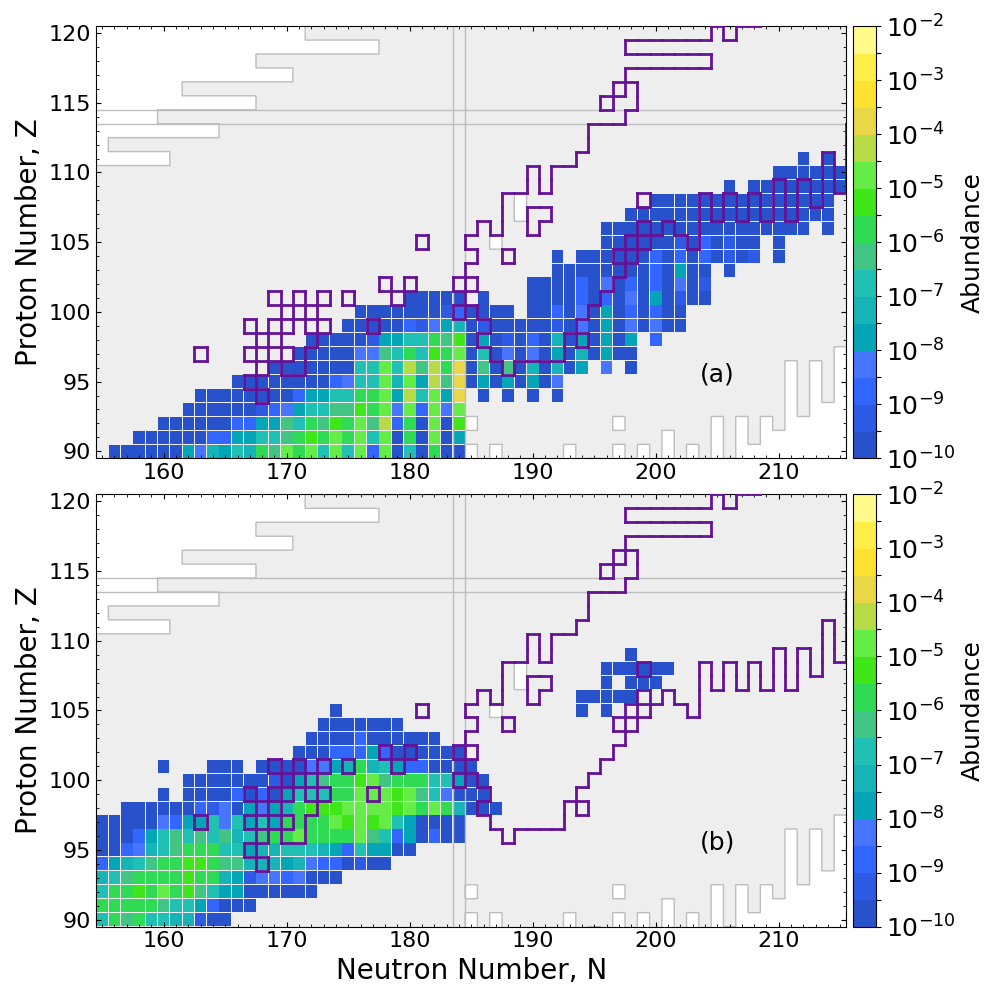Network calculation of tidal ejecta from a neutron star merger (FRDM2012)

$\beta$df alone prevents the production of superheavy elements in nature

Mumpower et al. ApJ 869 1 (2018) • Vassh et al. J. Phys. G (2019)

## Long-lived actinides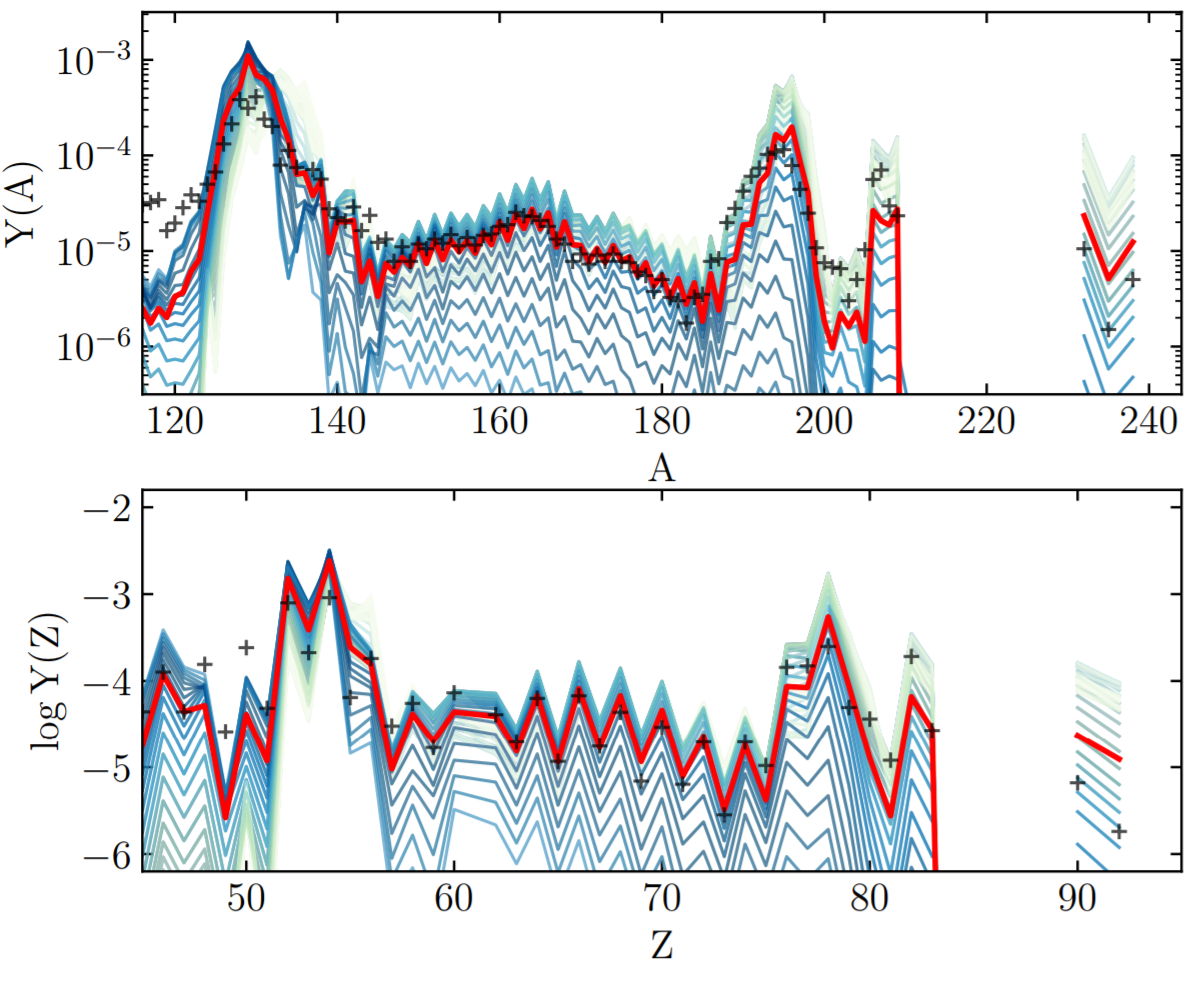With careful fission treatments: if actinides are produced, they are usually overproduced versus lanthanides

A sufficient amount of dilution with ligher $r$-process material is required to match the solar isotopic residuals

∴ Fission theory has implications for galactic chemical evolution, etc.

Holmbeck et al. ApJ 870 1 (2019) • Vassh et al. J. Phys. G (2019) • Holmbeck et al. submitted (2019)

## Observational Impact of Californium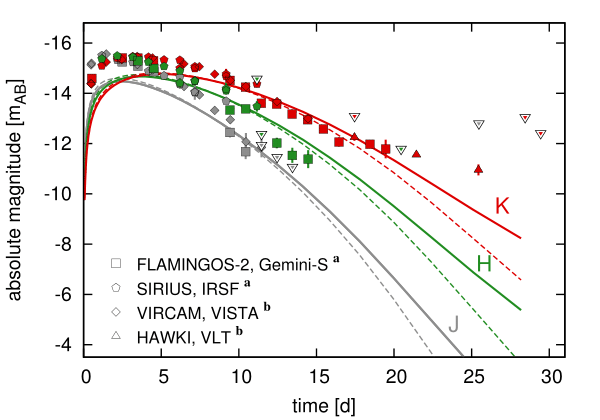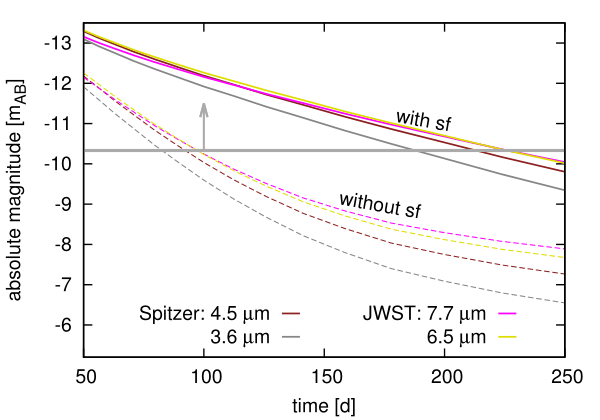Both near- and middle- IR are impacted by the presence of $^{254}$Cf

Late-time epoch brightness can be used as a proxy for actinide nucleosynthesis

Future JWST will be detectable out to 250 days with the presence of $^{254}$Cf

This also has implications for merger morphology...

Y. Zhu et al. ApJL 863 2 (2018) • Miller et al. in prep (2019) • Korobkin et al. in prep (2019)

## Calculated yield (Californium)Jaffke et al. PRC 67 034608 (2018) • Y. Zhu et al. ApJL 863 2 (2018) • Mumpower et al. in prep. (2019)

## FRLDM yields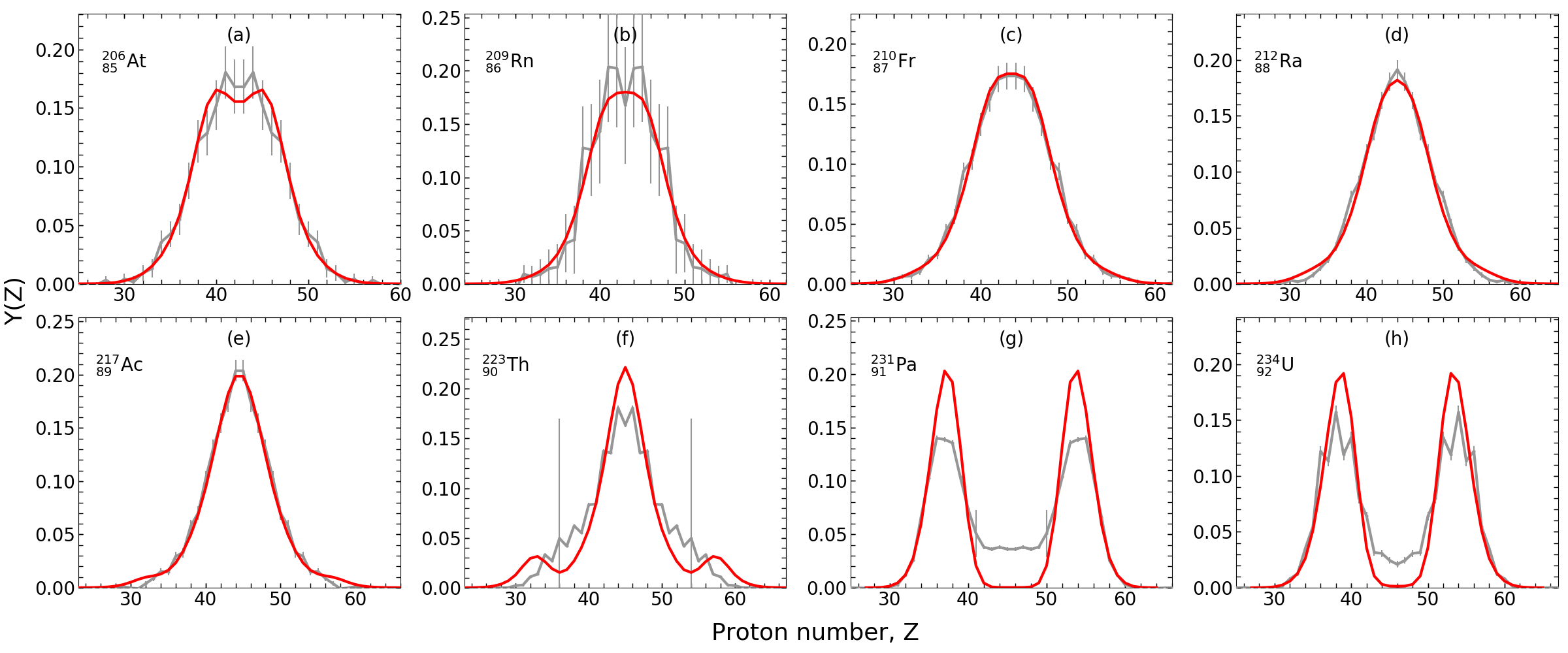Experiment Theory

FRLDM fragment yields have remarkable agreement with known data

Over a range of experiments, evaluations and nuclei!

Verriere & Mumpower in prep. (2019) • Mumpower et al. in prep. (2019)

## Special thanks to

My collaborators

A. Aprahamian, J. Barnes, B. Côté, J. Clark, C. Fryer, E. Holmbeck, P. Jaffke, T. Kawano, O. Korobkin, S. Liddick, G. C. McLaughlin, J. Miller, P. Möller, R. Orford, J. Randrup, G. Savard, A. Sierk, N. Schunck, T. Sprouse, A. Spyrou, I. Stetcu, R. Surman, P. Talou, N. Vassh, M. Verriere, R. Vogt, Y. Zhu
& many more...

Students Postdocs FIRE LANL

## Summary

The $r$-process relies on fission in many ways:

Re-cycling materialActinide productionLate-time observations

FRIB and other facilities will make a lot of measurements, but fission studies remain relatively inaccessible

Fission theory is crucial to understanding the formation of the heaviest elements (and $A\sim130$)

The FIRE Collaboration will soon provide a suite of new fission properties for the community:

Rates • Branchings • Yields • Q-values • Spectra

Results / Data / Papers @ MatthewMumpower.com

# Extra Slides

## How we calculate fission yields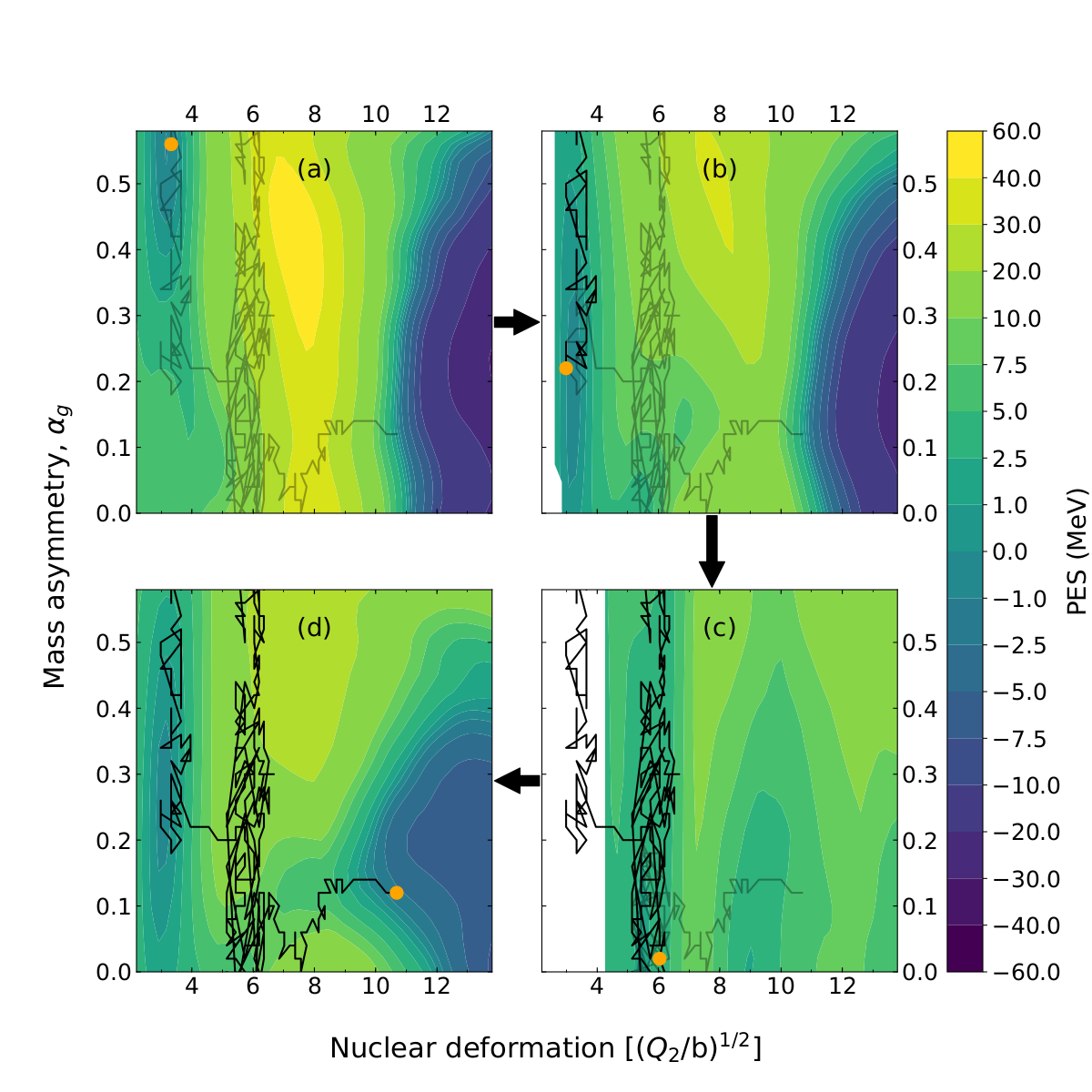We use a discrete random walk over a potential energy surface

This assumes strong disspative dynamics

The ensemble of such random walks produces the fission yield

Verriere & Mumpower in prep. (2019) • Mumpower et al. in prep. (2019)